# How to Correctly Use Math in Everyday Life

Math is not always overcomplicated. There are tricks that help ease the solving of any equation. You can learn to solve tasks that you come across in everyday life in faster and simpler ways.

We at 5-Minute Crafts collected simple life hacks that will help you quickly make calculations without using a calculator.

### How to learn quick mental addition. The first method.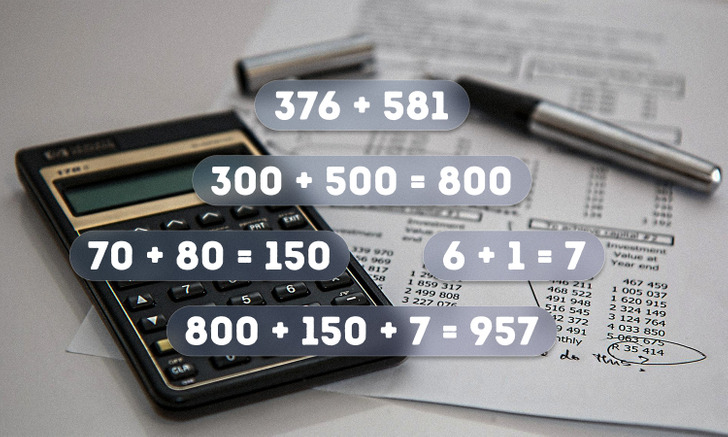To add large numbers quickly in your head, it is much more efficient to move from left to right than from right to left. For example, when adding 3-digit numbers, first add the hundreds, then the tens, ending with the ones.

All you need to do is to add the resulting 3 numbers and get the right result.

### How to learn quick mental addition. The second method.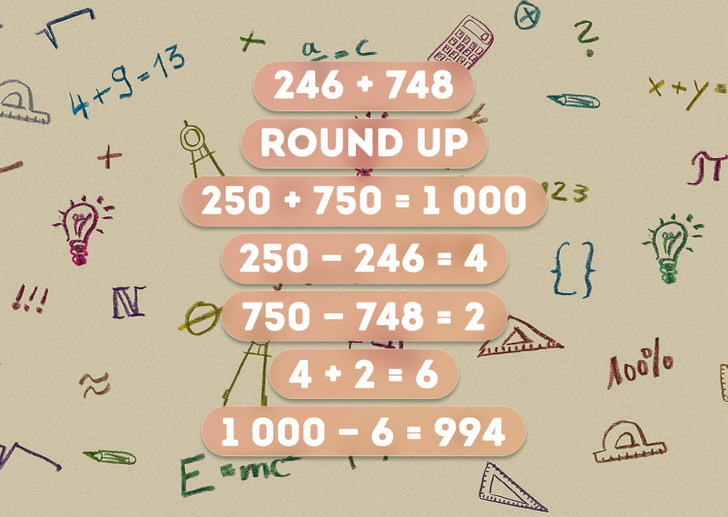To simplify mental addition, you need to round the terms by making them multiples of ten. The resulting numbers are then added up. To find the final answer, we must determine how much we added to the numbers to round them up. To do this, the original number is subtracted from the rounded number.

The resulting 2 numbers are then added up and the result is subtracted from the result of the first sum, which is the sum of rounded up numbers.

### How to quickly double any number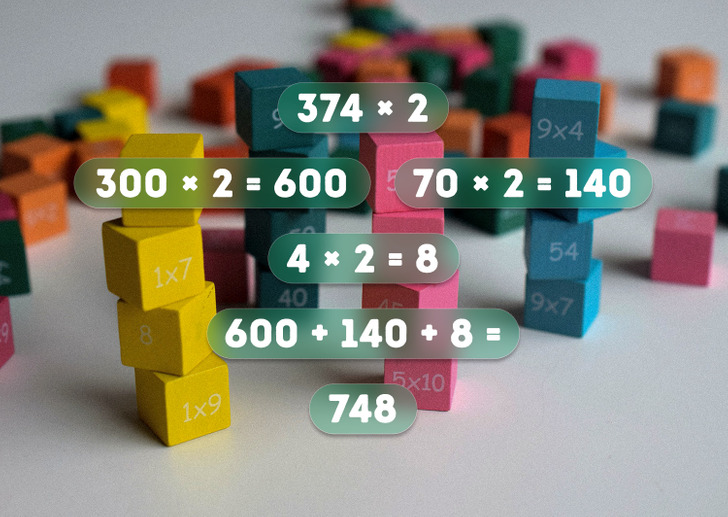To double a multi-digit number, you need to sequentially multiply the constituent numbers by 2, starting from the most significant digit. It is recommended to multiply numbers from left to right: this way it is easier to keep track of the results and not get confused.

In order to get the final result, the resulting numbers are added together.

### How to quickly subtract a number from 1,000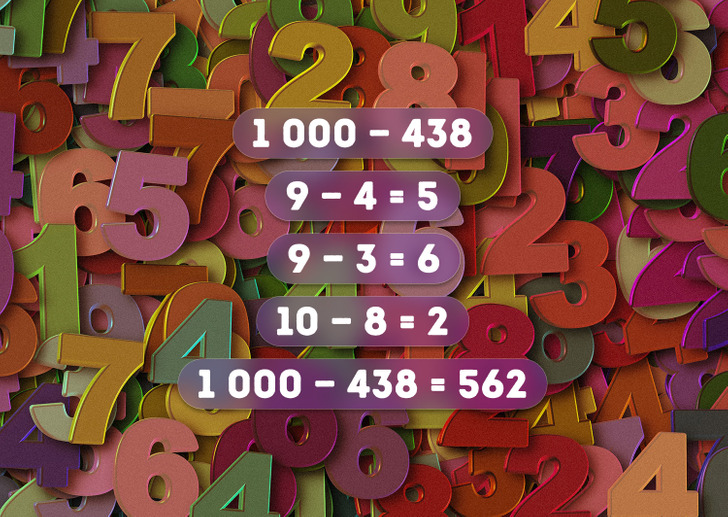To subtract a 3-digit number from 1,000, you need to sequentially subtract the numbers that make up each digit: hundreds and tens from 9, units from 10. The numbers obtained as a result of subtraction will form the hundreds, tens, and ones of the final result.

### How to easily multiply numbers ending with 0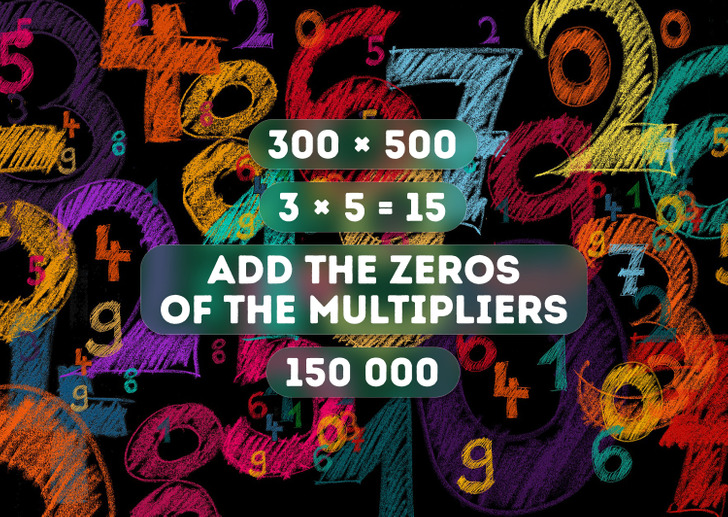simple way to multiply numbers that end in zero is to multiply the numbers preceding the zeros together, and then add all the zeros from the original multipliers to the result.

### How to calculate percents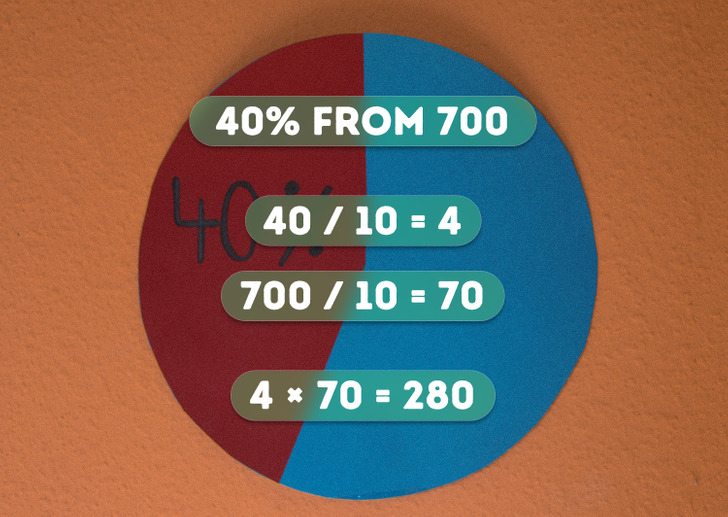Here is another way to easily find the percent of a number without a calculator. This way is suitable for finding any percent of a number.

For both numbers: the number of percent and the number the percent of which we are finding are divided by 10. Then multiply the products together to get your answer.

5-Minute Crafts/Tricks/How to Correctly Use Math in Everyday Life